# Fourier method of designing digital filters

Written by Paul Bourke
March 1999

### Introduction

The following describes the completely general method for designing digital filters, in this case we will restrict ourselves to causal (non recursive) filters. That is, filters of the formWhere x(i) in the input series, y(i) is the filtered series, bk are known as the filter coefficients of the filter of width W.

Broadly the technique involves creating the desired frequency response in the frequency domain, inverse filtering to get the impulse response (time domain), truncating the impulse response and optionally windowing it to reduce artefacts (Gibbs' phenomenon).

### Example

The following example will be presented to illustrate the technique for a low pass filter. While ideal low pass, high pass, and band pass filters (among others) can be derived analytically, the method discussed here applies to any filter for which the desired frequency response can be created.

The aim is to create a digital filter with a cut-off frequency of 50Hz. The sampling frequency will be assumed to be 500Hz, although this is only relevant for interpretation of the frequency and time scales. The ideal low pass filter has a frequency magnitude response that is unity from 0 to 50Hz and zero elsewhere. The phase response will be discussed later but will be set to zero now, when the filter is made causal the filter will have a linear phase response.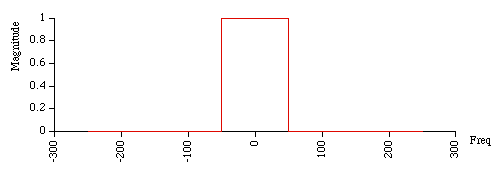If this frequency response is inverse Fourier transformed using a Fast Fourier Transform say, the result will be the impulse response of the filter in the time domain. It should come as no surprise that this is a sinc function centered at the origin.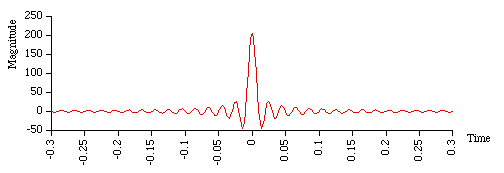There are a number of problems using these coefficients as the filter. First, the filter is non causal (there are coefficients to the left of the time origin) as it needs to know future values of the function it is filtering. While this is sometimes possible it isn't if the filter is to act in real time. Secondly, the filter length is infinite, in other words in order to filter with the ideal rectangular frequency response requires an infinite length filter.

These two issues are addressed by truncating the filter coefficients and then shifting the filter to the right so that all coefficients occur at or after the origin of the time axis. For example, the following has been truncated to 40 filter coefficients and then shifted right by 20 time steps to make it causal.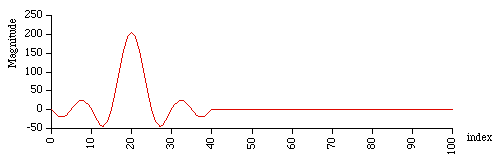As might be expected there is a penalty incurred for truncating the filter series to 40 coefficients, that penalty can be seen if you transform back into the frequency domain to view the new frequency response. Instead of the ideal rectangular response there is now "ringing" in the cut-off and the pass band, as well as a more gradual transition between the bands. The ripple is often called the Gibbs phenomenon after Willard Gibbs who documented it in 1899. That is life, there is no way to have a finite length (duration) filter and not have an infinite frequency response, the best one can do is minimise the effect.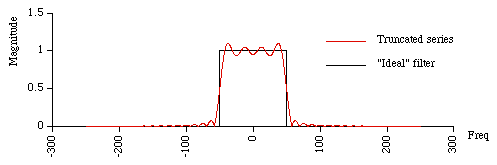There are two ways to reduce the departures from the ideal filter. The first is to increase the number of coefficients used by the filter. The following shows the frequency magnitude response for filter lengths of 20, 40, and 80. As expected, the transition becomes sharper as the filter order increases. The height of the ripple decreases but quite slowly. One disadvantage of using increasingly long filter lengths is the compute time required to perform the filtering.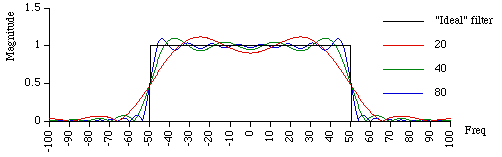The usual way to reduce the ripple is to window the impulse response in the time domain. Strictly speaking we were windowing the impulse response earlier but with a rectangular window. The effect was a convolution of the ideal frequency response with the appropriate sinc function. There are better windows that have a smoother transition in the time domain and reduced ripples in the frequency domain. The following shows the frequency response for filter lengths (width W) of 20, 40, and 80 using a Hanning window. This window is one of many but is a good trade-off between simplicity and side-band suppression. It is attributed to Julius von Hann and is sometimes called the raised cosine window that follows from its formula:

w(i) = 0.5 + 0.5 cos(2 pi i / W) for -W/2 <= i <= W/2 else w(i) = 0The ripples are much reduced although at the expense of the transition between the cut-off and the pass band. There are many other window types, for example the Bartlett window which is a triangular function and therefore corresponds to a convolution of the ideal frequency response by sinc2. A more sophisticated window with optimal characteristics (in a certain sense) is the Kaiser window.

The phase response is linear within the pass band due to the shifting in the time domain. One is generally not concerned with the phase response outside the pass band. The following is the phase response for a filter of width 40 for both the rectangular and Hanning windows.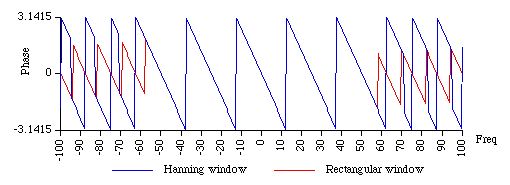It is traditional to display the magnitude response on a log10 scale, as follows for the three different width Hanning windowed example above.### Source code

This C source code (tab stops = 3) is provided as a basis for experimenting and creating digital filters based upon the techniques discussed above. It generates data files that permit ready plotting of the various stages of the process.